# MP Board Class 6th Maths Solutions Chapter 8 Decimals Ex 8.1

## MP Board Class 6th Maths Solutions Chapter 8 Decimals Ex 8.1

maths decimal rounding to the nearest hundredth ·

Question 1.
Write the following as numbers in the given table.Solution: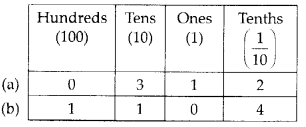When you round to 1 decimal place, you inspect the hundredths integer.

Question 2.
Write the following decimals in the place value table.
(a) 19.4
(b) 0.3
(c) 10.6
(d) 205.9
Solution: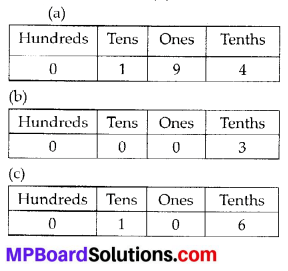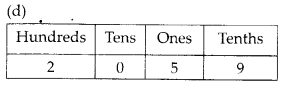What is 1/8 as a decimal? You can also take the fraction 3/4 and write it in highest terms to 75/100.

Question 3.
Write each of the following as decimals:
(a) Seven-tenths
(b) Two tens and nine-tenths
(c) Fourteen point six
(d) One hundred and two ones
(e) Six hundred point eight
Solution:
(a) Seven-tenths = 7 tenths = $$\frac{7}{10}$$ = 0.7
(b) Two tens and nine-tenths = $$2 \times 10+\frac{9}{10}$$
= 20 + 0.9 = 20.9
(c) Fourteen point six = 14.6
(d) One hundred and two-ones = 100 + 2 × 1
= 100 + 2 = 102
(e) Six hundred point eight = 600.8

Question 4.
Write each of the following as decimals: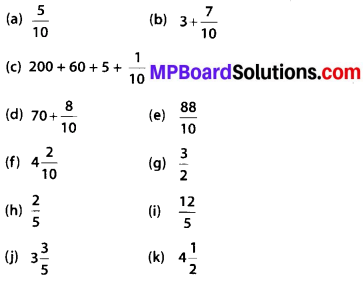Solution: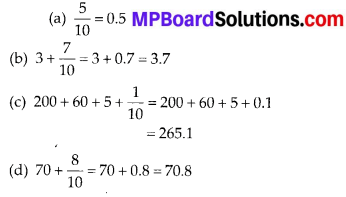To write 9/20 as a decimal you have to divide numerator by the denominator of the fraction.

Question 5.
Write the following decimals as fractions. Reduce the fractions to lowest form.
(a) 0.6
(b) 2.5
(c) 1.0
(d) 3.8
(e) 13.7
(f) 21.2
(g) 6.4
Solution: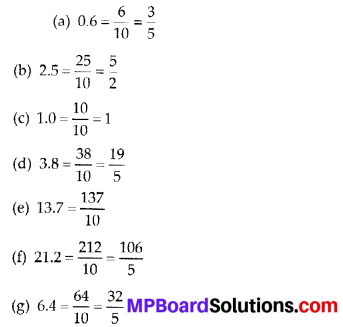Question 6.
Express the following as cm using decimals.
(a) 2 mm
(b) 30 mm
(c) 116 mm
(d) 4 cm 2 mm
(e) 162 mm
(f) 83 mm
Solution: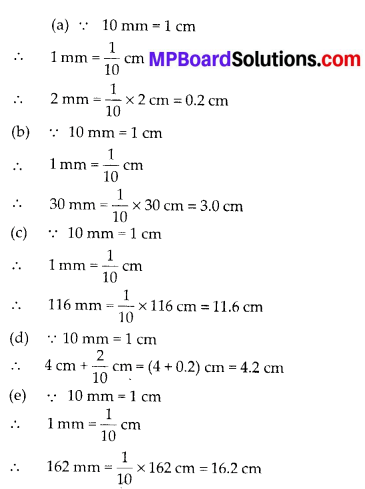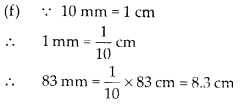Question 7.
Between which two whole numbers on the number line are the given numbers lie?
Which of these whole numbers is nearer the number?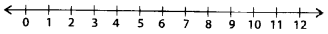Solution:
(a) 0.8
(b) 5.1
(C) 2.6
(d) 6.4
(e) 9.1
(f) 4.9
Solution:
(a) 0.8 lies between 0 and 1. From 0 to 1, 0.8 is nearer to 1
(b) 5.1 lies between 5 and 6. From 5 to 6, 5.1 is nearer to 5.
(c) 2.6 lies between 2 and 3. From 2 to 3, 2.6 is nearer to 3.
(d) 6.4 lies between 6 and 7. From 6 to 7, 6.4 is nearer to 6.
(e) 9.1 lies between 9 and 10. From 9 to 10, 9.1 is nearer to 9.
(f) 4.9 lies between 4 and 5. From 4 to 5, 4.9 is nearer to 5.Question 8.
Show the following numbers on the number line.
(a) 0.2
(b) 1.9
(c) 1.1
(d) 2.5
Solution:Question 9.
Write the decimal number represented by the points A, B, C, D on the given number line.Solution: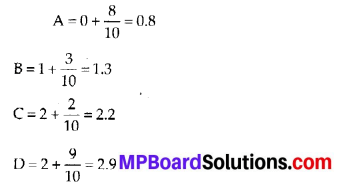Question 10.
(a) The length of Ramesh’s notebook is 9 cm 5 mm. What will be its length in cm?
(b) The length of a young gram plant is 65 mm. Express its length in cm.
Solution:
(a) 9 cm 5 mm = 9 cm + 5 mm
= $$\left(9+\frac{5}{10}\right)$$ cm = 9.5 cm

(b) 65 mm = $$\frac{65}{10}$$ cm = 6.5 cm